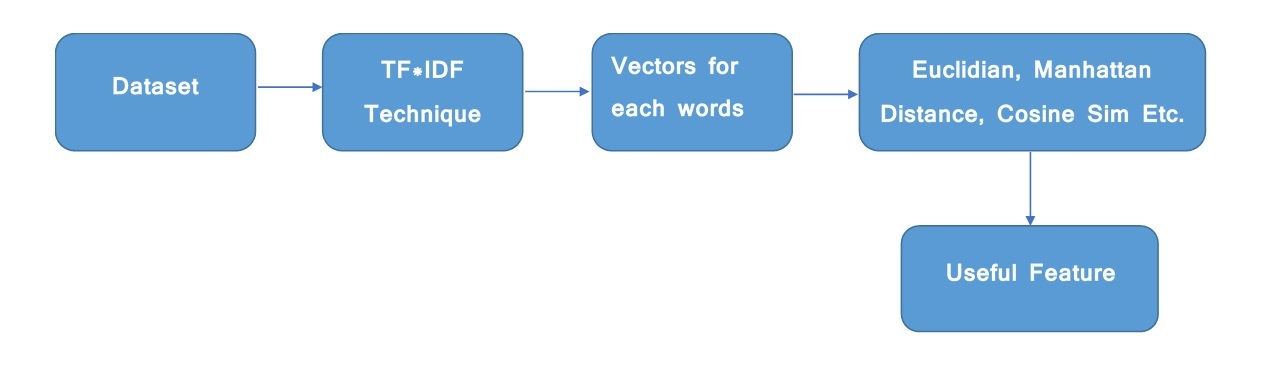# Sklearn | Feature Extraction with TF-IDF

Now, you are searching for tf-idf, then you may familiar with feature extraction and what it is. TF-IDF which stands for Term Frequency – Inverse Document Frequency. It is one of the most important techniques used for information retrieval to represent how important a specific word or phrase is to a given document. Let’s take an example, we have a string or Bag of Words (BOW) and we have to extract information from it, then we can use this approach.

The tf-idf value increases in proportion to the number of times a word appears in the document but is often offset by the frequency of the word in the corpus, which helps to adjust with respect to the fact that some words appear more frequently in general.
TF-IDF use two statistical methods, first is Term Frequency and the other is Inverse Document Frequency. Term frequency refers to the total number of times a given term t appears in the document doc against (per) the total number of all words in the document and The inverse document frequency measure of how much information the word provides. It measures the weight of a given word in the entire document. IDF show how common or rare a given word is across all documents.
TF-IDF can be computed as tf * idfTf*Idf do not convert directly raw data into useful features. Firstly, it converts raw strings or dataset into vectors and each word has its own vector. Then we’ll use a particular technique for retrieving the feature like Cosine Similarity which works on vectors, etc. As we know, we can’t directly pass the string to our model. So, tf*idf provides numeric values of the entire document for us.
To extract features from a document of words, we import –

`from sklearn.feature_extraction.text import TfidfVectorizer`

Input :

```1st Sentence - "hello i am pulkit"
2nd Sentence - "your name is akshit"
```

Code : Python code to find the similarity measures

 `# importing libraries ` `from` `sklearn.feature_extraction.text ``import` `TfidfVectorizer ` `from` `sklearn.metrics.pairwise ``import` `cosine_similarity ` `from` `sklearn.metrics ``import` `pairwise_distances ` `from` `sklearn.metrics.pairwise ``import` `euclidean_distances ` `from` `scipy.spatial ``import` `distance ` `import` `pandas as pd ` `import` `numpy as np ` `  `  `## Converting 3D array of array into 1D array ` `def` `arr_convert_1d(arr): ` `    ``arr ``=` `np.array(arr) ` `    ``arr ``=` `np.concatenate( arr, axis``=``0` `) ` `    ``arr ``=` `np.concatenate( arr, axis``=``0` `) ` `    ``return` `arr ` `  `  `## Cosine Similarity ` `cos ``=` `[] ` `def` `cosine(trans): ` `    ``cos.append(cosine_similarity(trans[``0``], trans[``1``])) ` `  `  `## Manhatten Distance ` `manhatten ``=` `[] ` `def` `manhatten_distance(trans): ` `    ``manhatten.append(pairwise_distances(trans[``0``], trans[``1``],  ` `                                        ``metric ``=` `'manhattan'``)) ` `  `  `## Euclidean Distance ` `euclidean ``=` `[] ` `def` `euclidean_function(vectors): ` `    ``euc``=``euclidean_distances(vectors[``0``], vectors[``1``]) ` `    ``euclidean.append(euc) ` `  `  `# This Function finds the similarity between two  ` `# sentences by using above functions. ` ` `  `## TF - IDF ` `def` `tfidf(str1, str2): ` `    ``ques ``=` `[] ` `    ``# You have to provide the dataset. Link of the dataset  ` `    ``# is given in the end of this article.  ` `    ``# and if you are using a different dataset then adjust  ` `    ``# it according to your dataset's columns and rows ` `    ``dataset ``=` `pd.read_csv(``'C:\\Users\\dell\\Desktop\\quora_duplicate_questions.tsv'``,  ` `                          ``delimiter``=``'\t'``,encoding``=``'utf-8'``) ` `     `  `    ``x ``=` `dataset.iloc[:, ``1``:``5``] ` `    ``x ``=` `x.dropna(how ``=` `'any'``) ` `     `  `    ``for` `k ``in` `range``(``len``(x)): ` `        ``for` `j ``in` `[``2``, ``3``]: ` `            ``ques.append(x.iloc[k, j]) ` `    ``vect ``=` `TfidfVectorizer() ` `    ``# Fit the your whole dataset. After all, this'll  ` `    ``# produce the vectors which is based on words in corpus/dataset ` `    ``vect.fit(ques) ` `  `  `    ``corpus ``=` `[str1,str2] ` `    ``trans ``=` `vect.transform(corpus) ` `  `  `    ``euclidean_function(trans) ` `    ``cosine(trans) ` `    ``manhatten_distance(trans) ` `    ``return` `convert() ` `  `  `def` `convert(): ` `    ``dataf ``=` `pd.DataFrame() ` `    ``lis2 ``=` `arr_convert_1d(manhatten) ` `    ``dataf[``'manhatten'``] ``=` `lis2 ` `    ``lis2 ``=` `arr_convert_1d(cos) ` `    ``dataf[``'cos_sim'``] ``=` `lis2 ` `    ``lis2 ``=` `arr_convert_1d(euclidean) ` `    ``dataf[``'euclidean'``] ``=` `lis2 ` `    ``return` `dataf ` `  `  `newData ``=` `pd.DataFrame();  ` `str1 ``=` `"hello i am pulkit"` `str2 ``=` `"your name is akshit"` `newData ``=` `tfidf(str1,str2); ` `print``(newData); `

Output :

```
manhatten  cos_sim  euclidean
0   2.955813      0.0   1.414214
```

Note: Dataset is large so it’ll take 30-40 second to produce output and If you are going to run as it is, then it’s not gonna work. It only works when you copy this code in your IDE and provide your dataset in tfidf function.

My Personal Notes arrow_drop_upCheck out this Author's contributed articles.

If you like GeeksforGeeks and would like to contribute, you can also write an article using contribute.geeksforgeeks.org or mail your article to contribute@geeksforgeeks.org. See your article appearing on the GeeksforGeeks main page and help other Geeks.

Please Improve this article if you find anything incorrect by clicking on the "Improve Article" button below.

Improved By : nidhi_biet